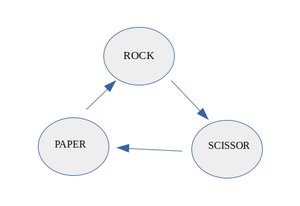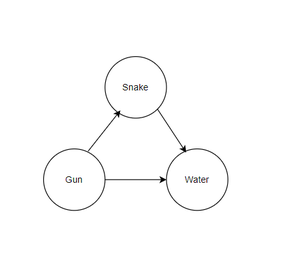Open in App
Not now

# Check whether the structure of given Graph is same as the game of Rock-Paper-Scissor

• Last Updated : 27 Jun, 2022

Given an array arr[] of N pairs of strings representing a directed graph, where each pair of the strings represents an edge between the nodes represented by the pair of strings, the task is to check whether the structure of the given directed graph is the same as that of the game of Rock-Paper-Scissor. If found true, then print “Yes“. Otherwise, print “No“.

Note:

In the real game of Rock-Paper-Scissor, the rock dominates over the scissor, scissor dominates over the paper and paper dominates over the rock.The game can be modelled using a directed graph as below, in which the directed edge (a, b) shows that a dominates over b.Examples:

Input: arr[] = {{“Snake”, “Water”}, {“Water”, “Gun”}, {“Gun”, “Snake”}}
Output: Yes
Explanation:Therefore, from the above image it can be seen that the given graph structure is similar to Rock-Paper-Scissor.

Input: arr[]={{“Snake”, “Water”}, {“Water”, “Gun”}, {“Gun”, “Gun”}}
Output: No

Approach: The problem can be solved by using the indegree and outdegree of the graphs based on the observation that in the directed graph Rock-Paper-Scissor there are only three nodes and indegree and outdegree of each node is one. Follow the steps below to solve the problem.

• Initialize two maps of {strings, int} say inDegree and outDegree, to store the indegree and outdegree of each node in these maps.
• Initialize a set of strings say st and insert all the nodes i.e. strings in this set.
• Check if the size of any map is not equal to 3 or the size of the set st of nodes is not equal to 3, then print “No“.
• Iterate over both the maps and check if the second value of any entry map is not 1, then, print “No” and break.
• Finally, if none of the above cases satisfy, then print “Yes“.

Below is the implementation of the above approach:

## C++

 `// C++ program for the above approach``#include ``using` `namespace` `std;` `// Function to check if the given directed``// graph has same structure to Rock-Paper-Scissor``string similarGame(vector > arr)``{` `    ``// Stores indegree of each node``    ``unordered_map indegree;` `    ``// Stores outdegree of each node``    ``unordered_map outdegree;` `    ``// Stores all nodes``    ``set st;` `    ``// Traverse through the edges``    ``for` `(``int` `i = 0; i < arr.size(); i++) {` `        ``// Check presence of self loop``        ``if` `(arr[i] == arr[i])``            ``return` `"No"``;` `        ``// Insert the node arr[i] and arr[i]``        ``// into the set``        ``st.insert(arr[i]);``        ``st.insert(arr[i]);` `        ``// Increment the outdegree and indegree of``        ``// nodes arr[i] and arr[i]` `        ``outdegree[arr[i]]++;``        ``indegree[arr[i]]++;``    ``}` `    ``// Check base conditions``    ``if` `(outdegree.size() != 3 || indegree.size() != 3``        ``|| st.size() != 3)``        ``return` `"No"``;` `    ``// Traverse the array outdegree``    ``for` `(``auto` `it : outdegree) {``        ``if` `(it.second != 1)``            ``return` `"No"``;``    ``}` `    ``// Traverse the array indegree``    ``for` `(``auto` `it : indegree) {``        ``if` `(it.second != 1)``            ``return` `"No"``;``    ``}` `    ``// Return``    ``return` `"Yes"``;``}` `// Driver Code``int` `main()``{` `    ``// Given Input``    ``vector > arr = { { ``"Snake"``, ``"Water"` `},``                                    ``{ ``"Water"``, ``"Gun"` `},``                                    ``{ ``"Gun"``, ``"Snake"` `} };` `    ``// Function Call``    ``cout << similarGame(arr);` `    ``return` `0;``}`

## Python3

 `# Python 3 program for the above approach` `# Function to check if the given directed``# graph has same structure to Rock-Paper-Scissor``def` `similarGame(arr):``    ``# Stores indegree of each node``    ``indegree ``=` `{}` `    ``# Stores outdegree of each node``    ``outdegree ``=` `{}` `    ``# Stores all nodes``    ``st ``=` `set``()` `    ``# Traverse through the edges``    ``for` `i ``in` `range``(``len``(arr)):``        ``# Check presence of self loop``        ``if` `(arr[i][``0``] ``=``=` `arr[i][``1``]):``            ``return` `"No"` `        ``# Insert the node arr[i] and arr[i]``        ``# into the set``        ``st.add(arr[i][``0``])``        ``st.add(arr[i][``1``])` `        ``# Increment the outdegree and indegree of``        ``# nodes arr[i] and arr[i]``        ``if` `arr[i][``0``] ``in` `outdegree:``            ``outdegree[arr[i][``0``]] ``+``=` `1``        ``else``:``            ``outdegree[arr[i][``0``]] ``=` `0``        ``if` `arr[i][``1``] ``in` `indegree:``            ``indegree[arr[i][``1``]] ``+``=` `1``        ``else``:``            ``indegree[arr[i][``1``]] ``=` `0` `    ``# Check base conditions``    ``if` `(``len``(outdegree) !``=` `3` `and` `len``(indegree) !``=` `3` `and` `len``(st) !``=` `3``):``        ``return` `"No"``;` `    ``# Traverse the array outdegree``    ``for` `key,value ``in` `outdegree.items():``        ``if` `(value ``=``=` `1``):``            ``return` `"No"` `    ``# Traverse the array indegree``    ``for` `key,value ``in` `indegree.items():``        ``if` `(value ``=``=` `1``):``            ``return` `"No"` `    ``# Return``    ``return` `"Yes"` `# Driver Code``if` `__name__ ``=``=` `'__main__'``:``    ``# Given Input``    ``arr ``=` `[[``"Snake"``, ``"Water"``],[``"Water"``, ``"Gun"``],[``"Gun"``, ``"Snake"``]]` `    ``# Function Call``    ``print``(similarGame(arr))``    ` `    ``# This code is contributed by SURENDRA_GANGWAR.`

## Javascript

 ``

## Java

 `/*package whatever //do not write package name here */` `import` `java.io.*;``import` `java.util.*;` `class` `GFG {``    ``// Java program for the above approach` `// Function to check if the given directed``// graph has same structure to Rock-Paper-Scissor``static` `String similarGame(String[][] arr)``{` `    ``// Stores indegree of each node``    ``HashMap indegree = ``new` `HashMap<>();` `    ``// Stores outdegree of each node``    ``HashMap outdegree = ``new` `HashMap<>();` `    ``// Stores all nodes``    ``TreeSet st = ``new` `TreeSet<>();` `    ``// Traverse through the edges``    ``for` `(``int` `i = ``0``; i < arr.length; i++) {` `        ``// Check presence of self loop``        ``if` `(arr[i][``0``] == arr[i][``1``])``            ``return` `"No"``;` `        ``// Insert the node arr[i] and arr[i]``        ``// into the set``        ``st.add(arr[i][``0``]);``        ``st.add(arr[i][``1``]);` `        ``// Increment the outdegree and indegree of``        ``// nodes arr[i] and arr[i]` `        ``if``(outdegree.containsKey(arr[i][``0``])){``            ``outdegree.put(arr[i][``0``],outdegree.get(arr[i][``0``])+``1``);``        ``}``        ``else` `outdegree.put(arr[i][``0``],``1``);``        ``if``(indegree.containsKey(arr[i][``0``])){``            ``indegree.put(arr[i][``0``],indegree.get(arr[i][``0``])+``1``);``        ``}``        ``else` `indegree.put(arr[i][``0``],``1``);``    ``}` `    ``// Check base conditions``    ``if` `(outdegree.size() != ``3` `|| indegree.size() != ``3``        ``|| st.size() != ``3``)``        ``return` `"No"``;` `    ``// Traverse the array outdegree``    ``for` `(Map.Entry it : outdegree.entrySet()) {``        ``if` `((``int``)it.getValue() != ``1``)``            ``return` `"No"``;``    ``}` `    ``// Traverse the array indegree``    ``for` `(Map.Entry it : indegree.entrySet()) {``        ``if` `((``int``)it.getValue() != ``1``)``            ``return` `"No"``;``    ``}` `    ``// Return``    ``return` `"Yes"``;``}` `/* Driver program to test above function*/``public` `static` `void` `main(String args[])``{``    ``// Given Input``    ``String[][] arr = { { ``"Snake"``, ``"Water"` `},{ ``"Water"``, ``"Gun"` `},{ ``"Gun"``, ``"Snake"` `} };` `    ``// Function Call``    ``System.out.println(similarGame(arr));``}` `}` `// This Solution is contributed by shinjanpatra.`

Output

`Yes`

Time Complexity: O(N)
Auxiliary Space: O(N)

My Personal Notes arrow_drop_up